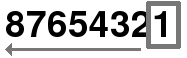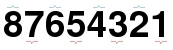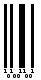:

# STANDARD 2 OF 5 BARCODE

## Format of the 2 of 5 standard

Length Type checksum
variable numeric optional

The standard 2 of 5 is variable length and includes an optional checksum digit 1

## Calculating the standard 2 of 5 checksum

Calculating the standard 2 of 5 checksum digit is very simple :

• The digit are used from right to left• Calculate the sum of the numbers of odd and even positions• Odd : 1 + 3 + 5 + 7 = 16
• Even : 2 + 4 + 6 + 8 = 20

### Standard 2 of 5 checksum formula :

( 10 - [ (3 * Odd + Even) modulo 10 ] ) modulo 10

Ainsi, l'exemple donne :

• (10 - [ (3 * 16 + 20) module 10 ] ) modulo 10
• (10 - [ (48 + 20) module 10 ] ) modulo 10
• (10 - [ (68) module 10 ] ) modulo 10
• (10 - [ 8 ] ) modulo 10
• (10 - 8) modulo 10
• 2 modulo 10
• 2

so the checksum of 87654321 est 2

### Standard 2 of 5 checksum generator :

Standard 2 of 5 message : Checksum :

## Binary sequence of standard 2 of 5 barcode

#### Start

A start sequence starts the barcode : 11011010

#### Message

Digit are encoded according to the following table :

digitsequence
0NNWWN
1WNNNW
2NWNNW
3WWNNN
4NNWNW
5WNWNN
6NWWNN
7NNNWW
8WNNWN
9NWNWN

"N" are encoded as "1" and "W" as "111", each one are separated by a "0", so the resulting table is :

digitsequence
010101110111010
111101010101110
210111010101110
311101110101010
410101110101110
511101011101010
610111011101010
710101011101110
811101010111010
910111010111010

#### Stop

A stop sequence ends the barcode : 11010110

## Graphical representation of the standard 2 of 5 barcode

Each element of the binary sequence is represented by a space of equal width, it is a "1" or "0". The "1" are represented by spaces filled and "0" with empty spaces.## Online standard 2 of 5 barcode generator

Please fill in the code :